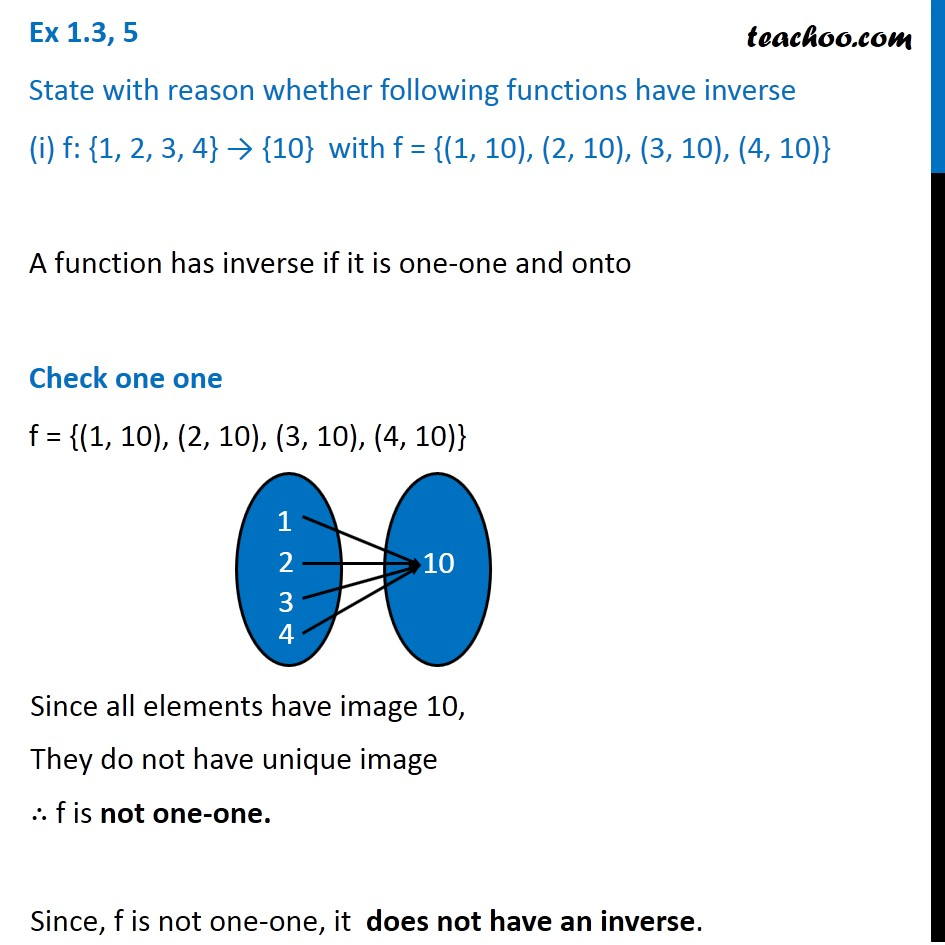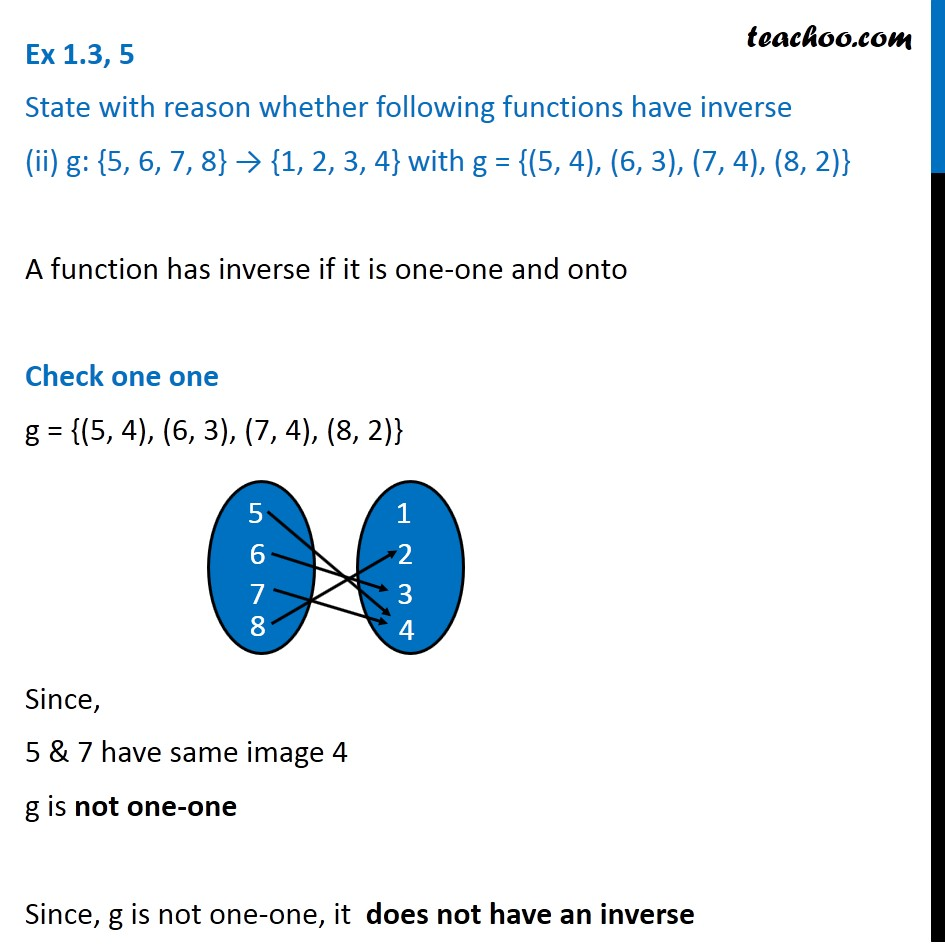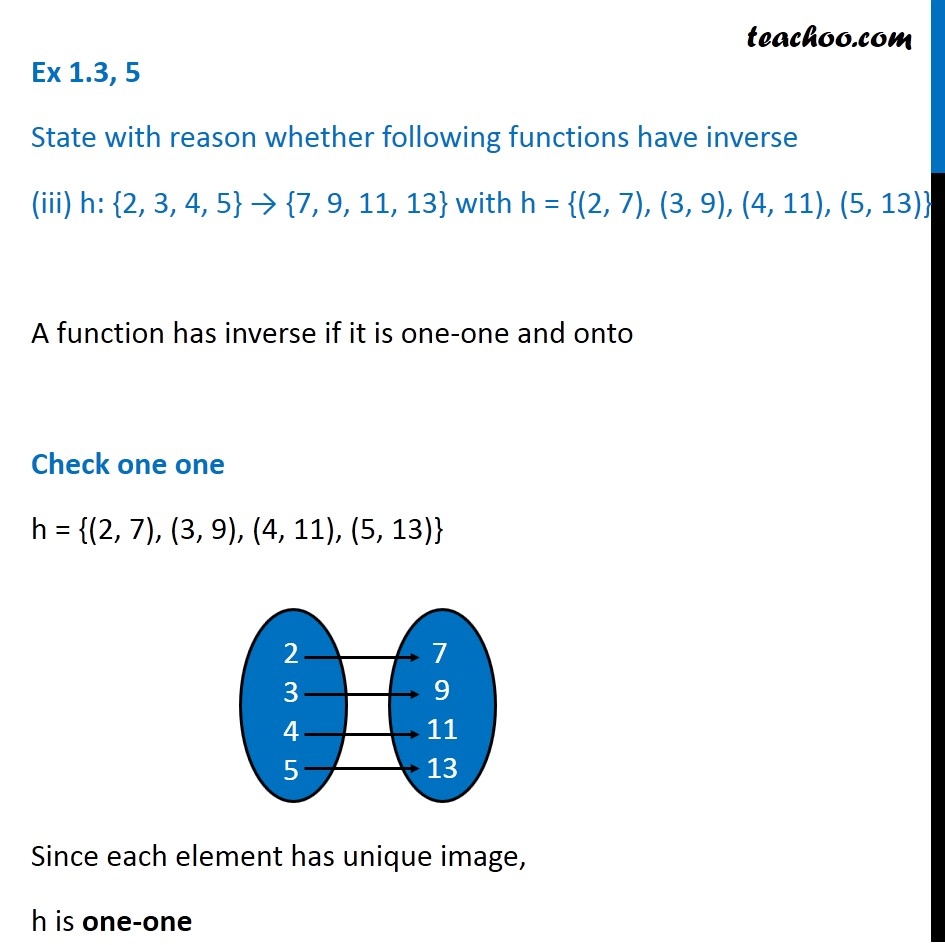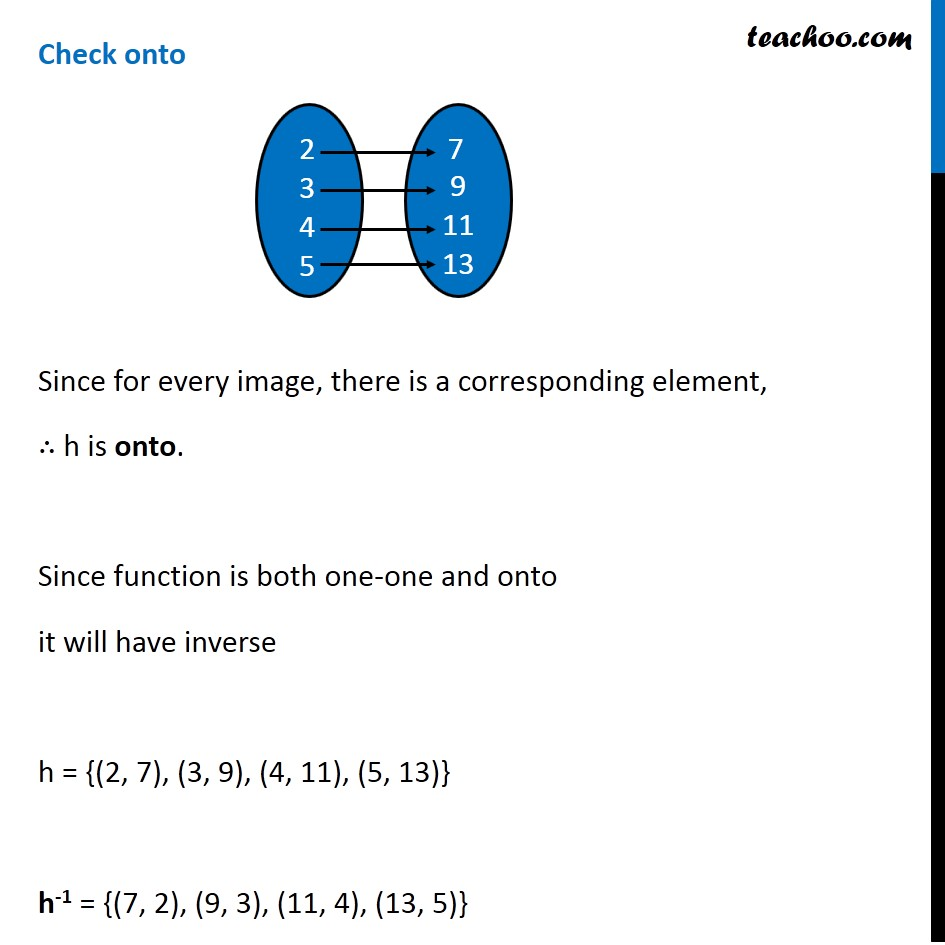Subscribe to our Youtube Channel - https://you.tube/teachoo

1. Chapter 1 Class 12 Relation and Functions
2. Serial order wise
3. Ex 1.3

Transcript

Ex 1.3, 5 State with reason whether following functions have inverse (i) f: {1, 2, 3, 4} → {10} with f = {(1, 10), (2, 10), (3, 10), (4, 10)} A function has inverse if it is one-one and onto Check one one f = {(1, 10), (2, 10), (3, 10), (4, 10)} Since all elements have image 10, They do not have unique image ∴ f is not one-one. Since, f is not one-one, it does not have an inverse. Ex 1.3, 5 State with reason whether following functions have inverse (ii) g: {5, 6, 7, 8} → {1, 2, 3, 4} with g = {(5, 4), (6, 3), (7, 4), (8, 2)} A function has inverse if it is one-one and onto Check one one g = {(5, 4), (6, 3), (7, 4), (8, 2)} Since, 5 & 7 have same image 4 g is not one-one Since, g is not one-one, it does not have an inverse Ex 1.3, 5 State with reason whether following functions have inverse (iii) h: {2, 3, 4, 5} → {7, 9, 11, 13} with h = {(2, 7), (3, 9), (4, 11), (5, 13)} A function has inverse if it is one-one and onto Check one one h = {(2, 7), (3, 9), (4, 11), (5, 13)} Since each element has unique image, h is one-one Check onto Since for every image, there is a corresponding element, ∴ h is onto. Since function is both one-one and onto it will have inverse h = {(2, 7), (3, 9), (4, 11), (5, 13)} h-1 = {(7, 2), (9, 3), (11, 4), (13, 5)}

Ex 1.3